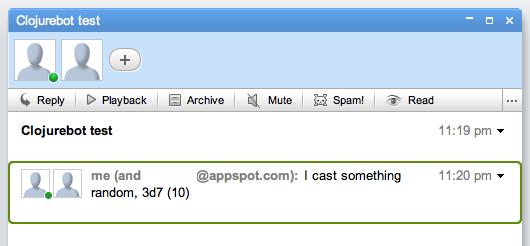# Building a Google Wave robot in Clojure

October 29, 2009

I’ve seen a few different posts circulating around on how to build a robot for Wave in Clojure, so I’ll throw mine in the pool.

I started off creating a text-replacement robot in Java, but decided to switch it over to Clojure and to make something semi useful. I ended up making a robot to calculate Dungeons & Dragons dice rolls (so something like ‘3d8′ means roll an 8-sided die 3 times and add the results). The idea being you could type something like:

“I cast Magic Missle, 2d6″

and this robot would replace it with the text:

“I cast Magic Missle, 2d6 (5)”

Where 5 is the number that was given. See screenshot below:So, let’s go through how to make this robot. I’m going to end up skipping most of the gritty details of getting the actual robot set up and refer you to this well-written post about the subject, be sure to follow all the instructions and you should have a usable clojure-bot for Google Wave. This post will cover a basic explanation of the (basic) code used for the actual robot.

Finished reading the other post and playing with the code? Cool, here’s what my `servlet.clj` looks like:

So, let’s go through each function and explain it.

```(ns bibly.servlet
(:import
[com.google.wave.api Blip Event EventType RobotMessageBundle TextView Wavelet])
(:require
))```

The (ns bibly.servlet) code puts us into the bibly.servlet namespace and inside that namespace, we’re doing 3 things:

1. Generating a class that extends Google’s AbstractRobotServlet class. (Exactly what you would do in order to build a robot in Java).
3. Requiring the parts of the clojure-contrib library that we need.

```(defn blip-submitted-events
[events]
(filter (fn [e] (= (EventType/BLIP_SUBMITTED) (.getType e))) events))```

This function (taken directly from spanx) is a filter that returns only the events that match EventType.BLIP_SUBMITTED.

```(defn roll-die
"Roll a die with  sides."
[sides]
(+ 1 (rand-int sides)))```

This function returns a dice roll (Integer) for a die with <sides> sides. (I removed the commented out line)

```(defn calculate-rolls
"Given a re-pattern match group, calculate the dice roll"
[txt]
(let [string (first txt)
dice (Integer. (second txt))
sides (Integer. (nth txt 2))]
(str string " (" (reduce + (take dice (repeatedly #(roll-die sides)))) ")")))```

Calualte-rolls is the main function for determining the roll, a vector is passed in, that looks like `["1d6" "1" "6"]`, this function then assigns each of the 3 values to a variable. Calculate-rolls then repeatedly calls `(roll-die sides)` dice times, reducing the list of results by adding them together. For example, if this function were passed [“2d4″ “2” “4”] , it would take a list of 2 (dice) rolls of a die with 4 (sides), and add them together to get the new value.

After reducing to the result integer, a string concatenating the result to the new string is returned. So for the example vector `["1d6" "1" "6"]` (roll a 6-sided die once), the result would be a string: `"1d6 (4)"`.

```(defn roll
"Given a text DnD dice roll, replace it with the calculated result."

(str-utils2/replace text (re-pattern #"(\d+)d(\d+)") calculate-rolls))```

The roll function takes a string containing a XdY statement, and expands the roll inside of the string.

```(defn replace-rolls
"Replace all the rolls in a given blip."
[blip]
(let [view (.getDocument blip)
text (.getText view)]
(.replace view (roll text))))```

Replace-rolls does the exact thing roll does for a string, just doing it for a given blip. First we get the document contained by a blip, and the text in that document, then replace it with the text that the `(roll "...")` function returns.

```(defn -processEvents
[this bundle]
(let [wavelet (.getWavelet bundle)]
(doseq [event (blip-submitted-events (.getEvents bundle))]
(replace-rolls (.getBlip event)))))```

Finally, the -processEvents function, which is the function google wave automatically calls when a capability matching those in capabilities.xml happens, is this function we perform the `(replace-rolls ...)` function on the list of events filtered by the `(blip-submitted-events ...)` function.

Well, I hope this example makes sense. Again, 95% of the work for this goes to Spanx‘s post for getting the initial setup, I figured it would be nice to have another example explaining the actual Clojure code for a simple robot’s inner workings.

You can download the code for this DnD robot from my github project, or Spanx’s censorbot example from his github project.

This example isn’t perfect, it needs a lot of work before it’s actually useful, but I thought it was neat.

tags: , , ,
posted in clojure, google, wave by Lee

### 1 Comment to "Building a Google Wave robot in Clojure"

1. […] a Google Wave robot in Clojure (here, via […]